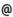## "Babes-Bolyai" University of Cluj-Napoca Faculty of Mathematics and Computer Science

 Mathematical economics
 Code Semes-ter Hours: C+S+L Credits Type Section ME277 1 2+1+1 7 compulsory Matematică Aplicată
 Teaching Staff in Charge
 Prof. PETRUSEL Adrian Olimpiu, Ph.D., petruselmath.ubbcluj.ro
 Aims To provide basic notions and main tools in the field of mathematical economics: multivalued analysis, maximal elements, eqilibrium points. To use these tools in the theory of equilibrium of an abstract economy.
 Content 1. Description of an economy. Arrow-Debreu model. 2. The analysis of multi-valued operators. 3. Fixed point, sstrict fixed point and coincidence point theorems for single-valued and multi-valued mappings. 3. KKM theory and variational inequalities. Optimal preferences. 4. Walrasian equilibrium of an economy. 5. Elements of game theory.
 References 1) J.W.S.CASSELS, Economics for mathematicians, Cambridge Univ.Press, 1989. 2) A.MAS-COLELL, An introduction to the differentiable approach in the theory of economic equilibrium, Univ.of California, Berkley, 1978. 3) A. PETRUSEL, Multivalued analysis and mathematical economics, House of the Book of Science, Cluj-Napoca, 2004. 4) J.P.AUBIN, Optima and Eqilibria, Springer, Berlin, 1993. 5) G.X.Z. YUAN, KKM Theory and Applications in Nonlinear Analysis, Marcel Dekker, New York, 1999. 6. K. BORDER, Fixed point theorems with applications to economic and game theory, Cambridge University Press, London, 1985. 7) A. PETRUSEL, Multifunctii si aplicatii, Presa Univ. Clujeana, Cluj-Napoca, 2001.
 Assessment Seminar activities (written test and a writing report) 40%, Final written test (at the end of the semester) 60%.